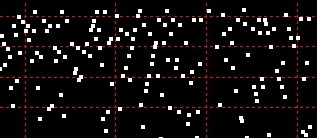Perfect Squareshome

 Lists of Perfect Squares and Perfect Square Generator with Roots and IntervalsView in your browser, download files, or generate your own 1 * 1 = 1 2 * 2 = 4 3 * 3 = 9 4 * 4 = 16 5 * 5 = 25 6 * 6 = 36 7 * 7 = 49 8 * 8 = 64 9 * 9 = 81 10 * 10 = 100 11 * 11 = 121 12 * 12 = 144 13 * 13 = 169 14 * 14 = 196 15 * 15 = 225 16 * 16 = 256 17 * 17 = 289 18 * 18 = 324 19 * 19 = 361 20 * 20 = 400 21 * 21 = 441 22 * 22 = 484 23 * 23 = 529 24 * 24 = 576 25 * 25 = 625 26 * 26 = 676 27 * 27 = 729 28 * 28 = 784 29 * 29 = 841 30 * 30 = 900 31 * 31 = 961 32 * 32 = 1024 33 * 33 = 1089 34 * 34 = 1156 35 * 35 = 1225 36 * 36 = 1296 37 * 37 = 1369 38 * 38 = 1444 39 * 39 = 1521 40 * 40 = 1600 41 * 41 = 1681 42 * 42 = 1764 43 * 43 = 1849 44 * 44 = 1936 45 * 45 = 2025 46 * 46 = 2116 47 * 47 = 2209 48 * 48 = 2304 49 * 49 = 2401 50 * 50 = 2500 These are comma-delimited text files containing 3 columns: - the square root (the counting number) - the perfect square (the square number) - the interval (the difference from the previous perfect square). First 100 1 - 10,000  (2KB) First 1,000 1 - 1,000,000  (23KB) First 10,000 1 - 100,000,000  (263KB) First 100,000 1 - 10,000,000,000 (2.94MB) First 1,000,000 1 - 1,000,000,000,000 (9.67MB - zip download only)   Want Even More Perfect Squares...? Generate lists of perfect squares up to 10^27! Output perfect squares in a selected range to text files. Save the program (PS-Gen.exe) to a folder. Specify From and To values (must be less than 10^15). Select the range (number of values*) for each text file. Click Start. What you get? The first number is the perfect square root (the counting number); the second number is the perfect square (square number); the third number is the interval from the previous perfect square. (For example, 101, 10201, 201 means 10201 is the 101st perfect square and the previous square number is 10000.) *If you accept the default of 100,000, that means 100,000 values (and lines) will be written to each file.